# Hydromagnetic Second-Order Fluid Flow in a Channel with Fluid -Particle Suspension and Viscous Dissipation

Hydromagnetic Second-Order Fluid Flow in a Channel with Fluid -Particle Suspension and Viscous Dissipation
Silchar Collegiate School, Silchar, Assam 788003, India

Corresponding Author Email:
hrd2910@gmail.com
Page:
1673-1679
|
DOI:
https://doi.org/10.18280/ijht.390532
31 July 2021
|
Accepted:
20 October 2021
|
Published:
31 October 2021
| Citation

OPEN ACCESS

Abstract:

This paper deals with the analysis of unsteady flow and heat transfer of second-order fluid with fluid-particle suspension in a vertical channel in the presence of transverse magnetic field with viscous dissipation. The fluid is driven by a constant pressure gradient. The governing partial differential equations are converted in to ordinary differential equation by similarity transformation and solved analytically. The expressions for velocity, temperature, skin friction and heat transfer are obtained. The numerical results depicting the effects of visco-elasticity in combination with other flow parameters involved in the problem are presented graphically and discussed qualitatively.

Keywords:

second-order fluid, fluid-particle suspension, shearing stress, visco-elasticity, heat transfer

1. Introduction

It is noticed that the linear relationship between stress and strain rate tensors unable to describe the rheology of industrial fluids, multi-phase mixture and biological fluids. The generalization of the one-dimensional constitutive equation led to the discovery of various types of non-Newtonian fluid flow. To describe the flow behaviour of these type of fluids several constitutive equations have been put forward under several rheological behaviour and visco-elastic fluid is one of them. Visco-elastic fluids exhibits two types of phenomena (a) viscosity of fluid and (b) elastic solid. Applications of visco-elastic fluid are observed in medical science, chemical industry and material processing. Due to its substantial demand significant interest have been developed and a large number of research papers have been contributed by various scientist and researchers [1-8].

Saffman  has proposed a dusty fluid model to analyse the stability of laminar flow of dusty gas. The flow of fluids with particle suspension finds its application in lubrication industry, combustion, petroleum industry, poly technology etc. Because of its wide range of application in industry significant interest have been developed on its utility in recent years and a large number of research papers have been contributed by various researchers [10-21]. In most of the cases, the fluid was taken to be Newtonian but from the practical point of view the study of non-Newtonian fluid is also important.

In the present analysis, hydromagnetic flow of visco-elastic fluid in a channel with fluid particle suspension along with viscous dissipation is considered. In this analysis, second order fluid model has been considered as it characterizes the visco-elastic fluid.

The constitutive equation of second-order fluid was put forward by Coleman and Markovitz  and Coleman and Noll  and is associated with dust as proposed by Saffman .

2. Mathematical Formulation

Consider the flow of conducting visco-elastic fluid with fluid particle suspension in vertical channel with radiative heat transfer. The vertical channel is filled with saturated porous medium. In the two-dimensional Cartesian coordinate system, x-axis lies along the centre of the channel and y-axis is in the normal direction. The vertical channel walls are located at y=0 and y=a. Also, the non-conducting channel walls are maintained at the temperature θ0 and θw. A uniform magnetic field of strength B0 is applied perpendicularly to the flow region.

It is assumed that fluid particle suspension is:

1. Solid, spherical and uniform in size.
2. Chemically inert, non-conducting and interaction between them are not considered.
3. Evenly distributed in the flow region and number density (N0) is fixed and maintained at uniform temperature in the flow region.

The governing equations are as follows:

\begin{align} & \frac{\partial \bar{u}}{\partial \bar{t}}=-\frac{1}{\rho }\frac{\partial P}{\partial \overset{\scriptscriptstyle\rightharpoonup}{x}}+{{v}_{1}}\frac{{{\partial }^{2}}\bar{u}}{\partial {{{\bar{y}}}^{2}}}+{{v}_{2}}\frac{{{\partial }^{3}}\bar{u}}{\partial \bar{t}\partial {{{\bar{y}}}^{2}}}-\frac{{{v}_{1}}}{K}\bar{u}+ \\ & \frac{{{N}_{0}}{{K}_{0}}}{\rho }({{{\bar{u}}}_{p}}-\bar{u})-\frac{{{\sigma }_{e}}B_{0}^{2}\bar{u}}{\rho }+g\beta (\theta -{{\theta }_{0}})\,\,\, \\ \end{align}                   (1)

$\frac{\partial {{{\bar{u}}}_{p}}}{\partial t}={{K}_{0}}(\bar{u}-{{\bar{u}}_{p}})$          (2)

\begin{align} & \frac{\partial \theta }{\partial \bar{t}}=\frac{k}{\rho {{c}_{p}}}\frac{{{\partial }^{2}}\theta }{\partial {{{\bar{y}}}^{2}}}-\frac{1}{\rho {{c}_{p}}}\frac{\partial q}{\partial \bar{y}}\,\,+\frac{{{U}^{2}}}{{{a}^{2}}}({{\mu }_{1}}{{\left( \frac{\partial \bar{u}}{\partial \bar{y}} \right)}^{2}} \\ & +{{\mu }_{2}}\frac{U}{a}\frac{\partial \bar{u}}{\partial \bar{y}}\frac{{{\partial }^{2}}\bar{u}}{\partial \bar{t}\partial \bar{y}})\,\, \\ \end{align}                         (3)

The initial boundary conditions are:

\left. \begin{align} & \bar{u}(y,0)={{{\bar{u}}}_{p}}(y,0)=0,\,\,\theta (y,0)={{\theta }_{f}}, \\ & \bar{u}(a,t)={{{\bar{u}}}_{p}}(y,0)=0,\,\, \\ & \theta (a,t)={{\theta }_{w}}={{\theta }_{0}}+({{\theta }_{f}}-{{\theta }_{0}}){{e}^{i\omega t}}, \\ & \bar{u}(0,t)={{{\bar{u}}}_{p}}(0,t)=0,\,\,\theta (0,t)={{\theta }_{0}}, \\ \end{align} \right\}\,                     (4)

where, u, up - velocities of fluid and dust particles in the x- direction, $\bar{t}$- time, ω - frequency of oscillation, θ, θf - fluid temperature and the initial fluid temperature, θ0, θw -the left and right wall temperature, P - fluid pressure, g- acceleration due to gravity, q- radiative heat flux, β- coefficient of volume expansion, K0- Stokes constant, D - average radius of dust particles, cp- specific heat at constant pressure, k- thermal conductivity, K- permeability porous medium, σe - conductivity of the fluid, ρ - fluid density, $v_{i}=\frac{\mu_{i}}{\rho}$ where i=1, 2, μe is the magnetic permeability and Ho is the intensity of magnetic field.

## 1.png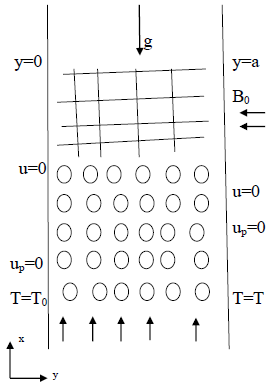Figure 1. Physical description of the problem

The fluid is assumed to be optically thin with a relatively low density and the radiative heat flux is given by Cogley et al. ,

$\frac{\partial q}{\partial t}=4{{\alpha }^{2}}({{\theta }_{0}}-\theta )$          (5)

where, α is the mean radiation absorption constant.

We introduce the following dimensionless variables:

\begin{align} & \bar{x}=\frac{x}{a},\bar{y}=\frac{y}{a},\bar{u}=\frac{u}{U},T=\frac{\theta -{{\theta }_{0}}}{{{\theta }_{f}}-{{\theta }_{0}}},\bar{t}=\frac{tU}{a},Da=\frac{K}{a{}^{2}}, \\ & M=\frac{\nu }{{{K}_{0}}{{a}^{2}}},l=\frac{{{N}_{0}}{{K}_{0}}{{a}^{2}}}{\rho \nu },\operatorname{Re}=\frac{Ua}{\nu },d=\frac{{{\nu }_{2}}U}{a{{\nu }_{1}}}, \\ & \Pr =\frac{v\rho {{c}_{p}}}{k},{{N}^{2}}=\frac{4{{\alpha }^{2}}{{a}^{2}}}{k},{{{\bar{u}}}_{p}}=\frac{{{u}_{p}}}{U},{{s}^{2}}=\frac{1}{Da} \\ & Gr=\frac{g\beta (T-{{T}_{0}}){{a}^{2}}}{vU},\bar{P}=\frac{aP}{v\rho U},{{H}^{2}}=\frac{{{a}^{2}}{{\sigma }_{e}}B_{0}^{2}}{v\rho }, \\ \end{align}

where, U is the velocity of the mean flow and d is the visco-elastic parameter.

The dimensionless governing equations together with the appropriate boundary conditions can be written as:

\begin{align} & \operatorname{Re}\frac{\partial u}{\partial t}=-\frac{\partial P}{\partial x}+\frac{{{\partial }^{2}}u}{\partial {{y}^{2}}}+d\frac{{{\partial }^{3}}u}{\partial t\partial {{y}^{2}}} \\ & -({{s}^{2}}+{{H}^{2}}+l)u+l{{u}_{p}}+Gr\theta \\ \end{align}                     (6)

$\operatorname{Re}M\frac{\partial {{u}_{p}}}{\partial t}=u-{{u}_{p}}$           (7)

\begin{align} & \operatorname{Re}\Pr \frac{\partial T}{\partial t}=\frac{{{\partial }^{2}}T}{\partial {{y}^{2}}}+{{N}^{2}}T\,+Ec\Pr ({{\left( \frac{\partial u}{\partial y} \right)}^{2}} \\ & +d\frac{\partial u}{\partial y}\frac{{{\partial }^{2}}u}{\partial t\partial y})\, \\ \end{align}                   (8)

The corresponding boundary conditions are

\left. \begin{align} & u(y,0)={{u}_{p}}(y,0)=0,\,\,\,\,T(y,0)=1,\,\, \\ & u(1,t)={{u}_{p}}(1,t)=0,\,\,\,\,T(1,t)={{e}^{i\omega t}},\,\, \\ & u(0,t)={{u}_{p}}(0,t)=0,\,\,\,\,T(0,t)=0 \\ \end{align} \right)               (9)

where, s - porous medium shape factor parameter, Da - Darcy number, Gr- Grashof number, H- Magnetic parameter, l- particle concentration parameter, M- particle mass parameter, N- radiation parameter, Re- flow Reynolds number and Pr - Prandtl number.

3. Method of Solution

In order to solve the Eqns. (6), (7) and (8) for pure oscillatory flow, let

\left. \begin{align} & -\frac{\partial P}{\partial x}=L{{e}^{i\omega t}},\,u(y,t)={{u}_{0}}{{e}^{i\omega t}}, \\ & {{u}_{p}}={{u}_{{{p}_{0}}}}{{e}^{i\omega t}},T(y,t)={{T}_{0}}{{e}^{i\omega t}} \\ \end{align} \right)               (10)

where, L- constant oscillation amplitude for pressure gradient.

On using the relation (10) in the Eqns. (6)-(8), we obtain:

$(1+di\omega )\frac{{{d}^{2}}{{u}_{0}}}{d{{y}^{2}}}-m_{2}^{2}{{u}_{0}}=-L-Gr{{T}_{0}}\,$         (11)

${{u}_{{{p}_{0}}}}=\frac{{{u}_{0}}}{(1+i\omega \operatorname{Re}M)}$         (12)

$\frac{{{d}^{2}}{{T}_{0}}}{d{{y}^{2}}}+m_{1}^{2}{{T}_{0}}=-Ec\Pr {{m}_{3}}{{e}^{i\omega t}}{{\left( \frac{\partial {{u}_{0}}}{\partial y}\, \right)}^{2}}$             (13)

The corresponding boundary conditions are

\left. \begin{align} & {{u}_{0}}={{u}_{{{p}_{0}}}}=0\,,\,\,\,\,\,\theta {}_{0}=0\,\,\,\,\,\,\,on\,\,\,y=0 \\ & {{u}_{0}}={{u}_{{{p}_{0}}}}=0\,,\,\,\,\,\,\theta {}_{0}=1\,\,\,\,\,\,\,\,on\,\,\,y=1\,\, \\ \end{align} \right)                       (14)

we assume

\begin{align} & {{u}_{0}}\left( y \right)={{u}_{1}}\left( y \right)+Ec{{u}_{2}}\left( y \right)+o{{\left( Ec \right)}^{2}} \\ & \,{{T}_{0}}\left( y \right)={{T}_{1}}\left( y \right)+Ec{{T}_{2}}\left( y \right)+o{{\left( Ec \right)}^{2}} \\ \end{align}

as Ec<<1.

We obtain the following sets of differential equations:

$m_{3}^{2}\frac{{{d}^{2}}{{u}_{1}}}{d{{y}^{2}}}-m_{2}^{2}{{u}_{1}}=-L-Gr{{T}_{1}}$          (15)

$m_{3}^{2}\frac{{{d}^{2}}{{u}_{2}}}{d{{y}^{2}}}-m_{2}^{2}{{u}_{2}}=-Gr{{T}_{2}}$         (16)

$\frac{{{d}^{2}}{{T}_{1}}}{d{{y}^{2}}}+m_{1}^{2}{{T}_{1}}=0$        (17)

$\frac{{{d}^{2}}{{T}_{2}}}{d{{y}^{2}}}+m_{1}^{2}{{T}_{2}}=-\Pr {{m}_{3}}{{e}^{i\omega t}}{{\left( \frac{\partial {{u}_{1}}}{\partial y}\, \right)}^{2}}\,\,$           (18)

The corresponding boundary conditions are:

\left. \begin{align} & {{u}_{1}}={{u}_{2}}={{T}_{1}}={{T}_{2}}=0\,\,\,\,\,\,\,\,\,\,\,\,on\,\,\,\,\,\,\,\,y=0 \\ & {{u}_{1}}={{u}_{2}}=0,{{T}_{1}}=1,\,\,{{T}_{2}}=0\,\,\,\,\,\,on\,\,\,\,\,\,y=1 \\ \end{align} \right)                (19)

Skin Friction (ST-1) for the dusty fluid at y=1 is given by:

$cf=\frac{{{\partial }^{2}}u}{\partial {{y}^{2}}}+d\frac{{{\partial }^{3}}u}{\partial t\partial {{y}^{2}}}$

and Skin Friction (ST-1) for the dusty fluid at y=1 is given by:

$Cf1=\frac{{{\partial }^{2}}{{u}_{p}}}{\partial {{y}^{2}}}+d\frac{{{\partial }^{3}}{{u}_{p}}}{\partial t\partial {{y}^{2}}}$

The coefficient of heat transfer in terms of Nusselt number (Nu) at y=1 is given by:

$Nu={{\left( \frac{\partial T}{\partial y} \right)}_{at\,y=0}}={{e}^{i\omega t}}{{\left( \frac{\partial {{T}_{0}}}{\partial y}+Ec\frac{\partial {{T}_{1}}}{\partial y} \right)}_{at\,y=0}}$

4. Results and Discussions

The main purpose of this work is to investigate the effect of visco-elastic parameter (d) in association with other flow parameters on hydromagnetic second order fluid flow in a vertical channel with fluid particle suspension and viscous dissipation. In order to get the physical insight of the problem the numerical values obtained for velocity of visco=elastic fluid (u), velocity of fluid particle suspension (up), temperature (T0), shearing stress of both visco-elastic (cf) and fluid particle suspension (cf1) and the rate of heat transfer (Nusselt number) are represented in graphically using MATLAB software. Few physical parameters as l=1; t=1; L=0.5; are remain fixed throughout the numerical calculation.

In the discussion for the nonzero valus of visco-elastic parameter (d) exhibits the flow of visco-elastic fluid (non-Newtonian fluid) otherwise it exhibits the flow of Newtonian fluid.

Figures 2-5 present the velocity profile of visco-elastic fluid (u) and fluid particle suspension (up).

Figures 2 and 3 show the effect of visco-elastic parameter on velocity of fluid (u) and particle suspension (up) in the channel. Figures 1 and 2 indicate that with the growth of visco-elastic parameter (d) reduces the speed of both visco-elastic fluid (u) and fluid particle suspension (up).

Figures 4 and 5 represent the influence of magnetic parameter (H) and Grashof number (Gr) on the velocity of visco-elastic fluid (u) and fluid particle suspension (up). When the strength of the applied magnetic field increases then the strength of Lorentz force which is resistive type of force also intensifies results in reduction of velocity of both visco-elastic fluid (u) and fluid particle suspension (up). On the other hand, Grashof number studies the process of free transmission of heat transfer. With the rise in Grashof number the buoyancy force also rises. The velocity of visco-elastic fluid (u) and fluid particle suspension (up) show a rising trend with the rise of Grashof number (Gr).

## 2.png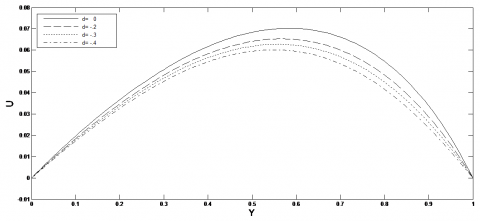Figure 2. Effect of visco-elastic parameter(d) on velocity profile u against y for Gr=4, H=5, Pr=6, Ec=0.04, M=0.2, Re=0.2, N=1, s=1, ω=1

## 3.png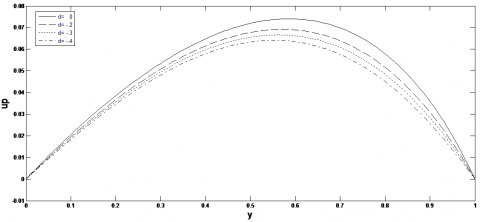Figure 3. Effect of visco-elastic parameter(d) on velocity profile up against y for Gr=4, H=5, Pr=6, Ec=0.04, M=0.2, Re=0.2, N=1, s=1, ω=1

## 4.pngFigure 4. Effect of Grashof number (Gr) and Magnetic parameter(H) on velocity profile u against y for Ec=0.04, M=0.2, Pr=6, Re=0.2, N=1, s=1, ω=1, d=-0.3

## 5.png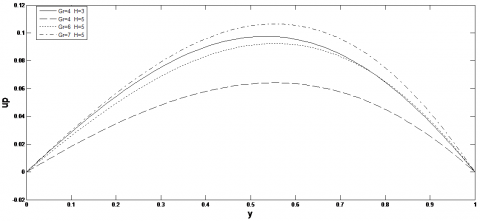Figure 5. Effect of Grashof number (Gr) and Magnetic parameter(H) on velocity profile up against y for Ec=0.04, M=0.2, Pr=6, Re=0.2, N=1, s=1, ω=1, d=-0.3

## 6.png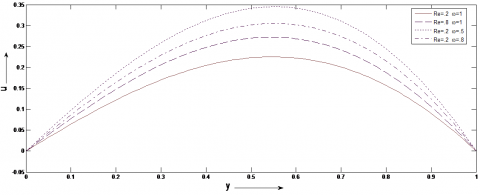Figure 6. Effect of Reynolds number (Re) and frequency of oscillation (ω) on velocity profile u against y for Ec=0.04, M=0.2, Pr=6, Gr=4, H=5, N=1, s=1, d=-0.3

## 7.png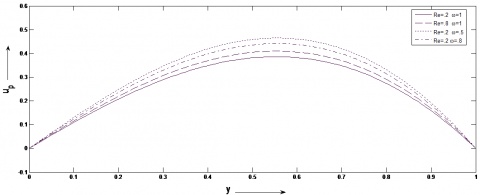Figure 7. Effect of Reynolds number (Re) and frequency of oscillation (ω) on velocity profile up against y for Ec=0.04, M=0.2, Gr=4, H=5, N=1, Pr=6, s=1, d=-0.3

Figures 6 and 7 represent the effect of Reynolds number (Re) and frequency of oscillation (ω) on the velocity of visco-elastic fluid (u) and fluid particle suspension (up). From these figures it is observed that with the rise of Reynolds number (Re) the intensity of viscous force will be less in governing in the flow of fluid and fluid particle suspension hence the velocity of visco-elastic fluid (u) and fluid particle suspension (up) also increases. But with the development of frequency of oscillation (ω) velocity of visco-elastic fluid (u) and fluid particle suspension (up) show a diminishing trend.

Figures 2-7, suggest that the velocity of visco-elastic fluid and fluid particle suspension (up) are maximum at y=0.5 i.e., at the centre of the channel.

Figures 8-11, presents the influence of visco-elastic parameter(d), Eckert number (Ec) and Grashof number (Gr) on temperature profile (T0) independent of time.

Figure 8, indicates that for increase in visco-elastic parameter (d) increases the temperature (T0) in the channel. Thus, it can be concluded that the temperature of visco-elastic fluid is amplified as compared to Newtonian fluid.

The effect of radiation parameter (N) on temperature (T0) is demonstrated in Figure 9. During the flow of visco-elastic fluid internal heat generation takes place accompanied by emission. This leads to the rise in temperature (T0) with the growth of radiation parameter (N).

## 8.pngFigure 8. Effect of visco-elastic parameter(d) on temperature(T0) against y for Gr=4, H=5, Pr=6, Ec=0.04, M=0.2, Re=0.2, N=1, s=1, ω=1

## 9.png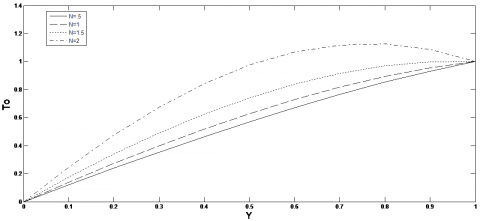Figure 9. Effect of radiation parameter (N) on temperature(T0) against y for Gr=4, H=5, Pr=6, Ec=0.04, M=0.2, Re=0.2, s=1, ω=1, d=-0.3

## 10.pngFigure 10. Effect of Eckert number (Ec) on temperature(T0) against y for Gr=4, H=5, M=0.2, Re=0.2, N=1, s=1, Pr=6, ω=1, d=-0.3

The influence of Eckert number (Ec) on temperature profile is exhibited in Figure 10. Due to rise in Eckert number (Ec) kinetic energy within the flow system also increases consequently. As a result, the temperature(T0) of the visco-elastic fluid also increases.

Also, it is noticed from the Figure 11 that increase in Grashof number (Gr) increases the temperature (T0) of the visco-elastic fluid.

Figures 12-15 represent the shearing stress of visco-elastic fluid and fluid particle suspension.

The effect of magnetic parameter (H) and radiation parameter (N) on the shearing stress of fluid phase (cf) and particle phase (cf1) are represented in Figures 12 and 13. During the growth of radiation parameter (N) the shearing stress of both visco-elastic fluid (cf) and fluid particle suspension (cf1) at the wall y=1 also show a rising trend. This may be regarded as there is a rise in velocity gradient of visco-elastic fluid along with fluid particle suspension at the channel wall. But with growth of magnetic parameter (H) shearing stress of both visco-elastic fluid and fluid particle suspension show a diminishing trend due to fall in velocity gradient at the channel wall.

## 11.png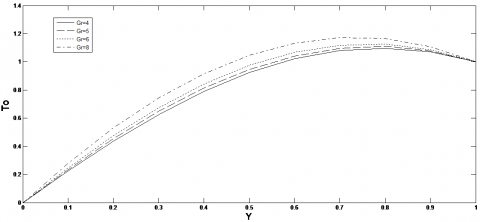Figure 11. Effect of Grashof number (Gr) on temperature(T0) against y for H=5, Ec=0.04, Pr=6, M=0.2, Re=0.2, N=1, s=1, ω=1, d=-0.3

## 12.png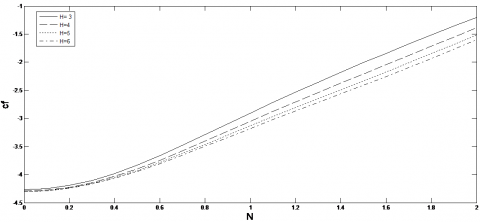Figure 12. Effect of magnetic parameter (H) and Radiation parameter(N) on shearing stress(cf) for Gr=4, Ec=0.04, M=0.2, Re=0.2, Pr=6, s=1, ω=1, d=-0.3

## 13.png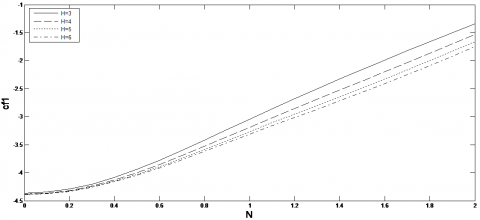Figure 13. Effect of magnetic parameter (H) and radiation parameter (N) on shearing stress(cf1) for Gr=4, Ec=0.04, Pr=6, M=0.2, Re=0.2, s=1, ω=1, d=-0.3

## 14.png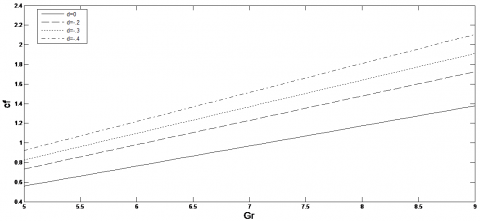Figure 14. Effect of Grashof number (Gr) and visco-elastic parameter(d) on shearing stress (cf) for H=5, Pr=6, Ec=0.04, M=0.2, Re=0.2, N=1, s=1, ω=1

## 15.png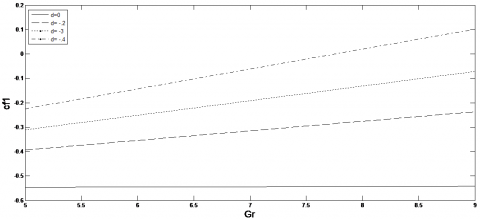Figure 15. Effect of Grashof number (Gr) and visco-elastic parameter(d) on shearing stress(cf1) for H=5, Ec=0.04, Pr=6, M=0.2, Re=0.2, N=1, s=1, ω=1

## 16.png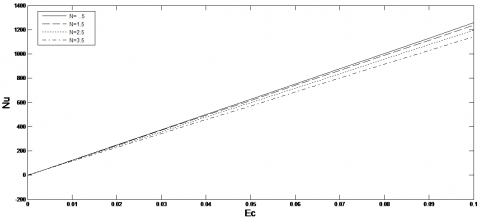Figure 16. Effect of radiation parameter (N) and Eckert number (Ec) on Nusselt number (Nu) for Gr=4, H=5, Pr=6, M=0.2, Re=0.2, s=1, ω=1, d=-0.3

## 17.png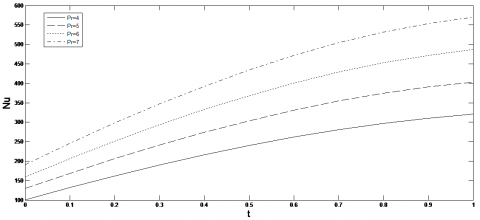Figure 17. Effect of Prandtl number (Pr) and time (t) on Nusselt number (Nu) for Gr=4, H=5, Ec=0.04, M=0.2, Re=0.2, N=1, s=1, ω=1, d=-3

Figures 14 and 15 describe the effects of Grashof number and visco-elastic parameter on visco-elastic fluid (cf) and fluid particle suspension (cf1) at the wall y=1. From these figures it can be concluded that rise in visco-elastic parameter (d) and buoyancy force in terms of Grashof number increases the shearing stress of both visco-elastic fluid (cf) and fluid particle suspension (cf1).

Figures 16 and 17 present the heat transfer in terms of Nusselt number.

The effect of dissipation parameter i,e, Eckert number (Ec) and radiation parameter (N) on the rate of heat transfer (Nusselt number) are observed in Figure 16. Due to growth of radiation parameter (N) there is a reduction in temperature gradient in the channel wall. Hence, the Nusselt number decreases with the rise of radiation parameter. Again, with the rise of dissipation parameter the internal heat generation capacity also increases. Thus, there is a growth in Nusselt number with the rise in Prandtl number.

The variation of Nusselt number (Nu) with time(t) and Prandtl number is observed in Figure 17. In heat transfer process for Prandtl number (Pr)>5 gives significance to momentum boundary layer than thermal boundary layer. So, due to increase in fluid momentum at the boundary surface the rate of heat transfer in terms of Nusselt number also increases with an increase in Prandtl number (Pr). Also, the rate of heat transfer increases with time (t).

5. Conclusions

In the present investigation, an effort has been made to observe the effects of thermal radiation, visco-elasticity, Lorentz force and buoyancy force on hydromagnetic flow of visco-elastic fluid in a channel with fluid particle suspension along with viscous dissipation. The results are obtained by perturbation technique for smaller values of Eckert number (Ec).

Some of the major findings are concluded below:

1. With the growth of magnetic parameter (H) and visco-elastic parameter (d), there is reduction in the velocity of visco-elastic-fluid and fluid particle suspension.
2. The velocity of visco-elastic-fluid and fluid particle suspension increases with the increase in Grashof number (Gr).
3. Temperature of visco-elastic fluid show a rising trend during the enhancement of visco-elastic parameter (d), Grashof number (Gr), Eckert number (Ec), radiation parameter (N).
4. Shearing stress of both visco-elastic-fluid and fluid particle suspension show a rising trend with the growth of Grashof number (Gr) and radiation parameter (N).
5. Shearing stress of both visco-elastic-fluid and fluid particle suspension show a declining trend with the growth of magnetic parameter (H) and visco-elastic parameter (d).
6. The rate of heat transfer (Nusselt number) shows an accelerating trend with the rise in Eckert number (Ec), Grashof number (Gr), Prandtl number (Pr) and time(t) but deaccelerating trend with the rise in radiation parameter (N).
References

 Abel, M.S., Siddheshwar, P.G., Nandeppanavar, M.M. (2007). Heat transfer in a viscoelastic boundary layer flow over a stretching sheet with viscous dissipation and non-uniform heat source. International Journal of Heat and Mass Transfer, 50(5-6): 960-966. https://doi.org/10.1016/j.ijheatmasstransfer.2006.08.010

 Talwar, K.K., Khomami, B. (1995). Flow of viscoelastic fluids pastperiodic square arrays of cylinders: Inertial and shear thinning viscosity and elasticity effects. J. of Non-Newtonian Fluid Mechanics, 57(2-3): 177-202. https://doi.org/10.1016/0377-0257(94)01290-X

 Rajagopal, K.R. (1984). Flow of visco-elastic fluid over a stretching sheet. Rheologica Acta, 23: 213-214. https://doi.org/10.1007/BF01332078

 Siddappa, B., Abel, S. (1985). Non-Newtonian flowpast a stretching plate. Zeitschrift f¨ur Angewandte Mathematik und Physik, 36(6): 890-892. https://doi.org/10.1007/BF00944900

 Anderson, H.I. (1992). MHD flow of a viscoelastic fluid past a stretching surface. Acta Mechanica, 95(1): 227-230. https://doi.org/10.1007/BF01170814

 Deka, B., Choudhury, R. (2018). On hydromagnetic flow of a second-grade fluid induced by an inclined plate. Int. J. of Heat and Technology, 36(1): 325-331. https://doi.org/10.18280/ijht.60143

 Rajakumar, K.V.B., Govinda Rao, T., Umasankara Reddy, M., Balamurugan, K.S. (2020). Influence of Dufour and thermal radiation on unsteady MHD Walter’s liquid model-B flow past an impulsively started infinite vertical plate embedded in a porous medium with chemical reaction, Hall and ion slip current. S.N Appl. Sci., 2: 742. https://doi.org/10.1007/s42452-020-2484-y742(2)

 Choudhury, R., Das, B. (2016). Influence of visco-elasticity on MHD heat and mass transfer flow through a porous medium bounded by an inclined surface with chemical reaction. International Journal of Heat and Technology, 34(2): 332-338. https://doi.org/10.18280/ijht.340225

 Saffman, P.G. (1962). On the stability of laminar flow of a dusty gas. J. Fluid Mech., 13(1): 120-129. https://doi.org/10.1017/S0022112062000555

 Gireesha, B.J., Roopa, G.S., Bagewadi, C.S. (2010). Unsteady flow and heat transfer of a dusty fluid through a rectangular channel. Hindawi Publishing Corporation, Mathematical Problems in Engineering, 2010: 898720. https://doi.org/10.1155/2010/898720

 Han, K.S., Sung, H.J., Chung, M.K. (1991). Analysis of heat transfer in a pipe carrying two-phase gas-particle suspension. Int. J. Heat and Mass Transfer, 34(1): 69-78. https://doi.org/10.1016/0017-9310(91)90174-D

 Dey, D. (2016). Non-Newtonian effects on hydromagnetic dusty stratified fluid flow through a porous medium with volume fraction. Proceedings of the National Academy of Sciences, India Section A: Physical Sciences, 86(1): 47-56. https://doi.org/10.1007/s40010-015-0230-4

 Khan, A.A., Tariq, H. (2020). Peristaltic flow of second-grade dusty fluid through a porous medium in an asymmetric channel. Journal of Porous Media, 23(9): 883-905. https://doi.org/10.1615/JPorMedia.2020024830

 Ali, F., Bilal, M., Gohar, M., Khan, I., Sheikh, N.A., Nisar, K.S. (2020). A report on fluctuating free convection flow of heat absorbing viscoelastic dusty fluid past in a horizontal channel with MHD effect. Scientific Reports, 10: 8523. https://doi.org/10.1038/s41598-020-65252-1

 Kulshretha, P.K., Puri, P. (1981). Wave structure in oscillatory Couette flow of a dusty gas. Acta Mech., 46: 127-128. https://doi.org/10.1007/bf01176769

 Makinde, O.D., Chinyoka, T. (2010). MHD transient flows and heat transfer of dusty fluid in a channel with variable physical properties and Navier slip condition. Comput. Math. Apl., 60(3): 660-669. https://doi.org/10.1016/j.camwa.2010.05.014

 Prakash, O.M., Makinde, O.D., Kumar, D., Dwivedi, Y.K. (2015). Heat transfer to MHD oscillatory dusty fluid flow in a channel filled with a porous medium. Sadhana, 40(4): 1273-1282. https://doi.org/10.1007/s12046-015-0371-9

 Attia, H.A., Al-Kaisy, A.M.A., Ewis, K.M. (2011). MHD Couette flow and heat transfer of a dusty fluid with exponential decaying pressure gradient. Tamkang J Sci Eng., 14(2): 91-96. https://doi.org/10.6180/jase.2011.14.2.01

 Kalita, B. (2012). Unsteady flow of a dusty conducting viscousliquid between two parallel plates in presence of a transverse magnetic field. Appl Math Sci., 6(76): 3759-3767.

 Gupta, R.K., Gupta, K. (1990). Unsteady flow of a dusty visco-elastic fluid through channel with volume fraction. Indian J Pure Appl Math., 21(7): 677-690.

 Ajadi, S.O. (2005). A note on the unsteady flow of dusty viscousfluid between two parallel plates. J Appl Math Comput., 18(1-2): 393-403. https://doi.org/10.1007/BF02936582

 Coleman, B.D., Markovitz, H. (1964). Incompressible second order fluid. Adv. Appl. Mech., 8: 69-101. https://doi.org/10.1016/S0065-2156(08)70353-3

 Coleman, B.D., Noll, W. (1960). An approximation theorem for functional with applications in continuum mechanics. Archs for Ration, Mech. and Analysis, 6: 355-370. https://doi.org/10.1007/BF00276168

 Cogley, A.C.L., Vinvent, W.C., Gilees, S.E. (1968). Differential approximation for radiative transfer in a nongrey gas near equilibrium. American Institute of Aeronautics and Astronautics, 6(3): 551. https://doi.org/10.2514/3.4538